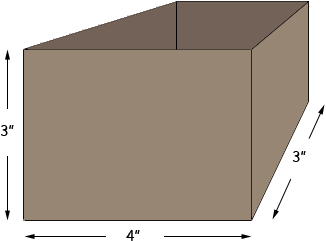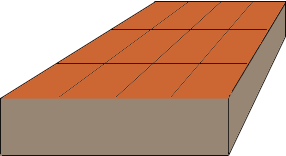Name: irene Who is asking: Parent Level of the question: Elementary Question: how do you find the volume in cubic units. i need the basic formula to help my child. Hi Irene, Suppose that you have a box that is 4 inches long, 3 inches wide and 3 inches high. You also have a collection of one inch cubes, that is wooden blocks that are 1 inch by 1 inch by 1 inch. The task is to see how many of these one inch cubes you can fit into the box. In other words how many cubic inches are in the box.Start by putting a layer of blocks on the bottom of the box. Since the box is 4 inches long you can place 4 blocks along the front edge of the box. Then you can place a second row of 4 blocks behind it and again another row of 4 blocks behind the second row.You have placed 43 = 12 blocks on the bottom layer of the box. The box is 3 inches high so you can put a second layer of 12 blocks on top of the first layer and then a third layer of 12 blocks to fill the box. Hence you have filled the box with 312 = 36, one cubic inch blocks. Hence the volume of the box is 36 cubic inches and you obtained the number 36 from 433 that is the length times the width times the height. This is the formula for the volume of a box volume = lengthwidthheight Penny